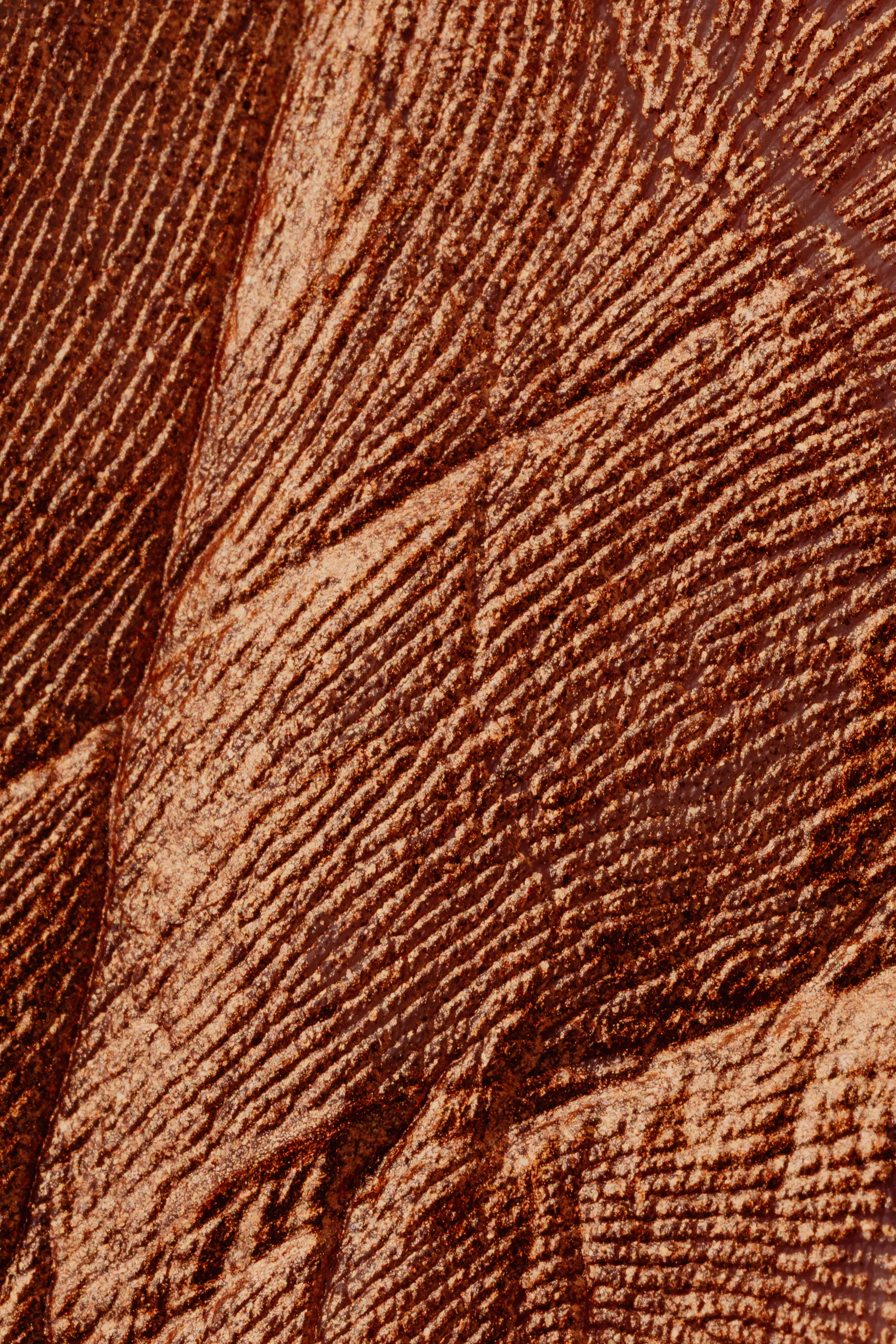# What is the hardest math class to take?

The Hardest Math Class to Take: Explained

# The Hardest Math Class to Take: Explained## Introduction

Mathematics is a subject that challenges the best of students. Some math courses are more challenging than others, and students often ask what is the hardest math class to take. In this article, we will explore the hardest math class in high school and college and help you understand why it is so challenging. By the end of this read, you’ll have a better understanding of what it takes to succeed in the hardest math class.

What is the hardest math class in high school?
Most high schools offer AP Calculus BC or IB Math HL as the hardest math course. AP Calculus BC covers the material in AP Calculus AB but also continues the curriculum, addressing more challenging and advanced concepts. Several factors make these courses difficult, including the speed at which the material is presented, the increased complexity of the concepts, and the high expectations of the students.
What is the hardest math class in college?
Several math classes are challenging in college, and choosing the hardest math class is subjective. Some universities consider Analysis, Algebra, or Topology courses to be the most challenging. These courses require not only a mastery of advanced math concepts but also the ability to think abstractly and logically.
What makes a math class hard?
The difficulty of a math class can be subjective because it depends on several factors, including the student’s background and experience, the teacher’s approach to teaching, and the curriculum’s rigor. However, some factors that contribute to making a math class hard include time constraints, challenging concepts, high expectations, and intense workload.
How can I prepare for the hardest math class?
Preparing for the hardest math class is challenging but doable. First, ensure that you have taken all the prerequisite math classes before the hardest math class. Review the material covered in previous classes and practice regularly. Attend tutoring sessions, seek help from peers or teachers, and participate in study groups. Understanding the fundamental concepts is crucial, so take your time and don’t rush through assignments or homework. Finally, don’t skip classes, take organized notes, and don’t be afraid to ask questions.

## The Hardest Math Class in High School

High school offers a wide range of math courses, and AP Calculus BC or IB Math HL is the most challenging. These classes are suitable for students who excel in math and are comfortable with problem-solving. The courses cover a wide range of concepts and take the students through an in-depth exploration of calculus, functions, and their applications. The volume of material can be overwhelming, and students must be committed to keeping up with the pace of the class.### AP Calculus BC

AP Calculus BC is a fast-paced, rigorous class that covers topics such as limits, derivatives, integrals, and Taylor series. The class moves more quickly than AP Calculus AB and requires a solid understanding of the fundamental concepts. Topics covered in BC that aren’t in AB include parametric equations, polar coordinates, vectors, and series. One of the most significant challenges of the class is the pace at which the material is presented. Students must keep up with the fast-paced nature of the course while also understanding the many complex concepts.

### IB Math HL

The International Baccalaureate (IB) Math HL course is another challenging course for high school students. The course covers topics such as calculus, algebra, geometry, statistics, and probability. The class requires fluency in mathematics as it moves quickly through advanced concepts. IB Math HL requires extensive problem-solving skills and the ability to work with abstract and theoretical concepts. The workload and expectations are high, and students are encouraged to work collaboratively and seek help from their teachers as needed.

## The Hardest Math Class in College

College math classes are much more in-depth and complex than high school courses. Several math courses are challenging, and students often have differing opinions on the hardest math class. Below are some of the college math courses considered the most challenging.### Analysis

Mathematical analysis is the study of limits, continuity, derivatives, integrals, sequences, and series. The course is considered challenging as it requires an abstract and theoretical approach to problem-solving. Students must think critically and logically to succeed in this course. It is also a prerequisite for several advanced math courses and is required for most majors in mathematics.

### Algebra

Algebra courses in college cover advanced abstract algebra concepts, group theory, matrices, fields, and rings. The course’s difficulty arises from the abstract nature of the concepts and the high expectations of the students. The course prepares students for other advanced math courses and is a focus for mathematics majors.

### Topology

Topology is the study of spaces and the properties that remain invariant under transformations such as bending, stretching, and squeezing. The course is considered challenging because it deals with abstract concepts and involves a lot of proofs. Students must have a solid understanding of mathematics foundations to succeed in topology.

## Conclusion

Math courses can be challenging, and determining the hardest math class is subjective. AP Calculus BC and IB Math HL are often regarded as the hardest math class in high school, while several classes such as Analysis, Algebra, or Topology are considered the most challenging in college. To succeed in the hardest math classes, students must be committed, have a strong foundation in mathematics, seek help from peers and teachers, and practice regularly. It may be challenging, but with proper preparation and dedication, any student can excel in even the most challenging math courses.